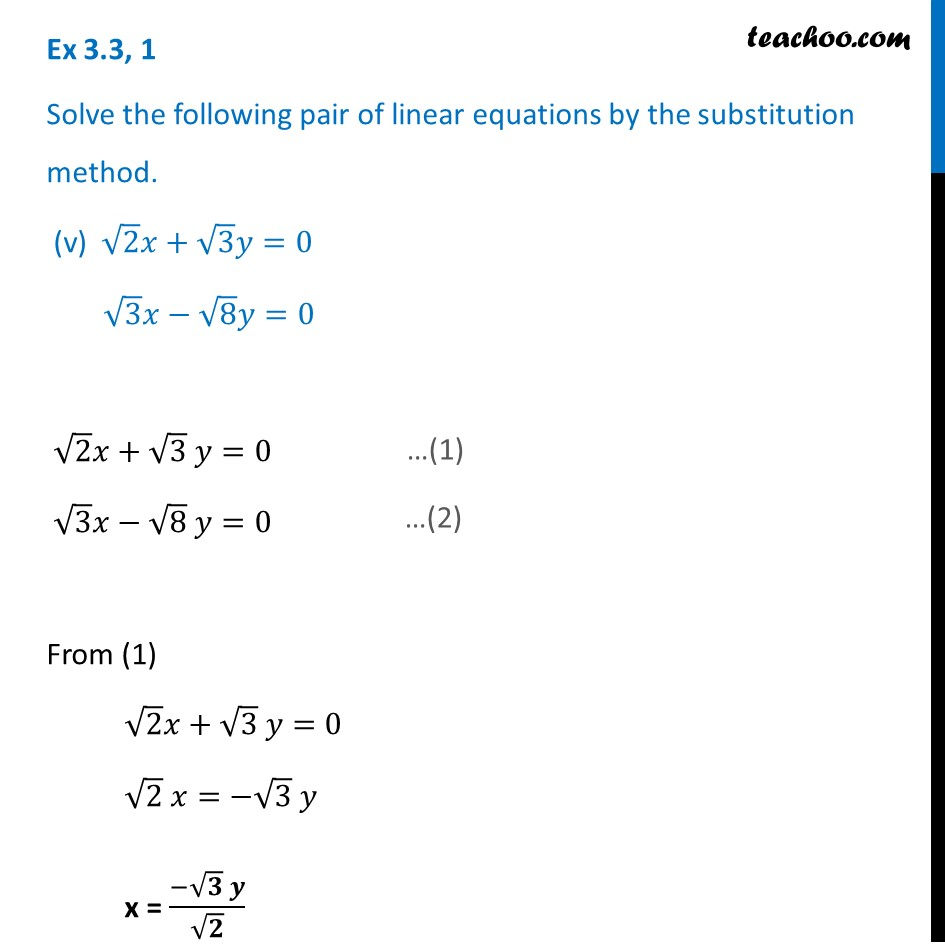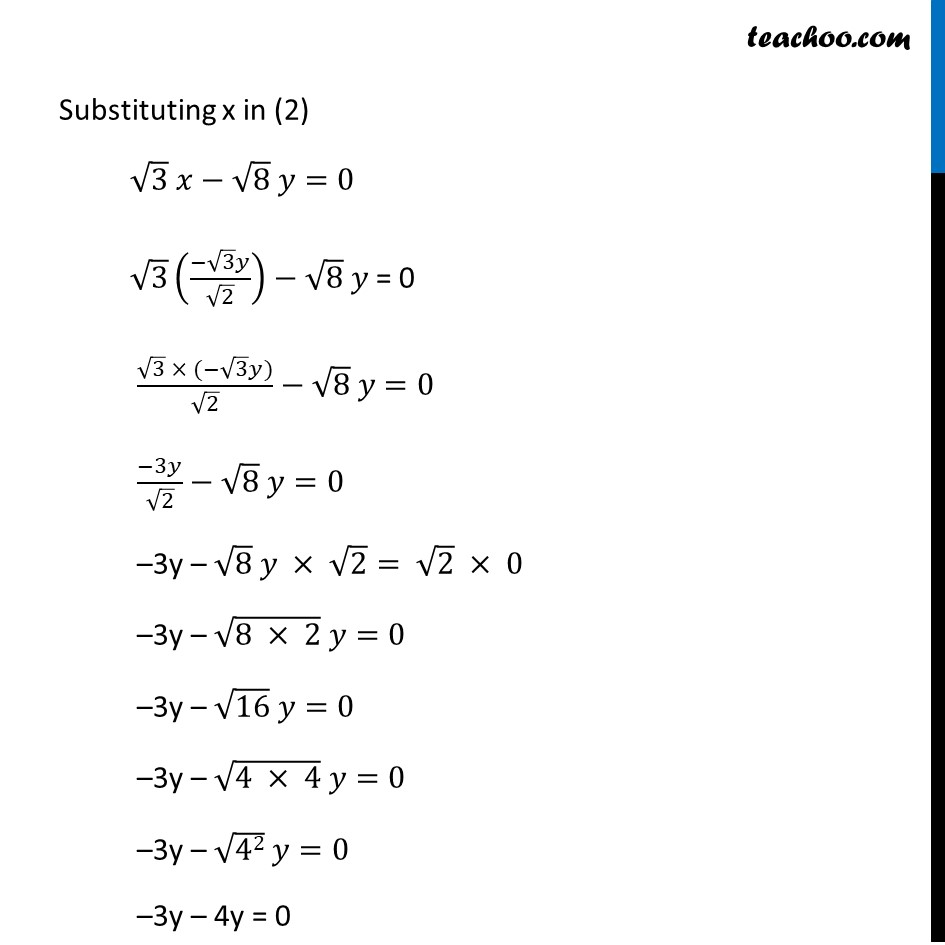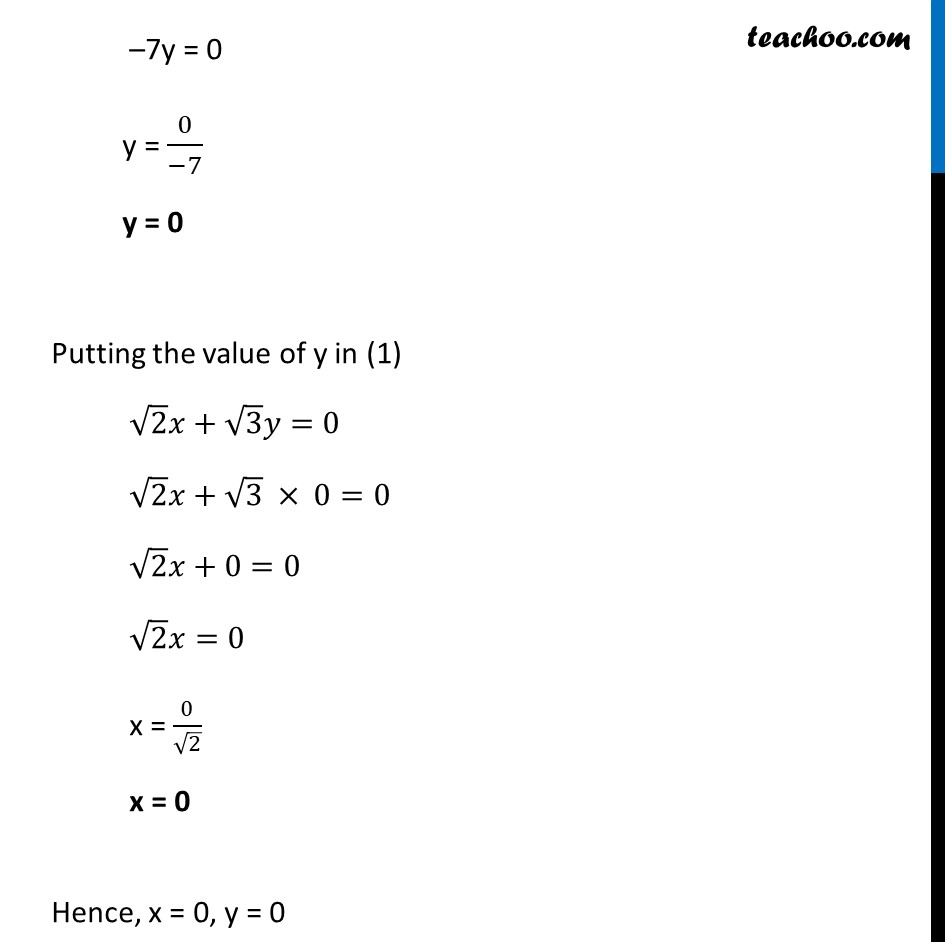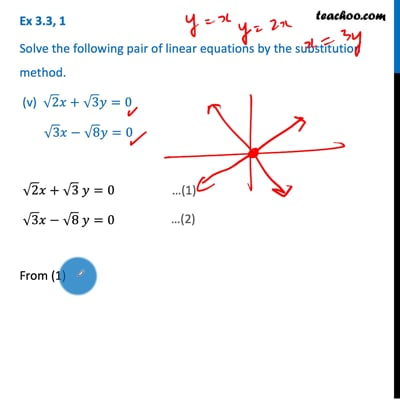Ex 3.3

Chapter 3 Class 10 Pair of Linear Equations in Two Variables
Serial order wiseThis video is only available for Teachoo black users

Get live Maths 1-on-1 Classs - Class 6 to 12

### Transcript

Ex 3.3, 1 Solve the following pair of linear equations by the substitution method. (v) √2 𝑥+√3 𝑦=0 √3 𝑥−√8 𝑦=0 √2 𝑥+√3 𝑦=0 √3 𝑥−√8 𝑦=0 From (1) √2 𝑥+√3 𝑦=0 √2 𝑥=−√3 𝑦 x = (−√𝟑 𝒚)/√𝟐 Substituting x in (2) √3 𝑥−√8 𝑦=0 √3 ((−√3 𝑦)/√2)−√8 𝑦 = 0 (√3 × (−√3 𝑦))/√2−√8 𝑦=0 (−3𝑦)/√2−√8 𝑦=0 –3y – √8 𝑦 × √2= √2 × 0 –3y – √(8 × 2) 𝑦=0 –3y – √16 𝑦=0 –3y – √(4 × 4) 𝑦=0 –3y – √42 𝑦=0 –3y – 4y = 0 –7y = 0 y = 0/(−7) y = 0 Putting the value of y in (1) √2 𝑥+√3 𝑦=0 √2 𝑥+√3 × 0=0 √2 𝑥+0=0 √2 𝑥=0 x = 0/√2 x = 0 Hence, x = 0, y = 0